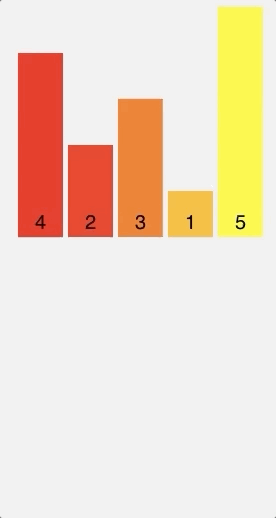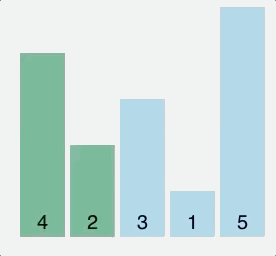# Sorting Algorithms with Javascript (Part 1)

October 18, 2018

I've been learning a lot about data structures and algorithms lately and I've noticed in my reading that there aren't a lot of examples showing implementations of algorithms in Javascript. You'll mostly find examples in Java, Python, C, C++ etc. Maybe there's a reason for preferring these languages over Javascript? I'm not sure.

In this first part, I'm going to show Javascript implementations of three sorting algorithms:

• Merge sort
• Insertion sort
• Bubble sort

This is not intended to be an in-depth explanation on the ins and outs of how the algorithms work and their performance. If you'd rather read about that, here's a nice resource I found: Sorting Algorithms

To keep things simple, I'll be sorting a simple list list having only 5 elements [4, 2, 3, 1, 5].

## Merge Sort

Merge sort uses a divide-and-conquer approach to sort elements in an array. Basically, what this means is that instead of dealing with the array as a whole, it continually splits it in half until both halves are sorted, then the halves are merged into one solved list.

#### Visual#### Code

function mergeSort(list) {
const len = list.length;
// an array of length == 1 is technically a sorted list
if (len == 1) {
return list;
}

// get mid item
const middleIndex = Math.ceil(len / 2);

// split current list into two: left and right list
let leftList = list.slice(0, middleIndex);
let rightList = list.slice(middleIndex, len);

leftList = mergeSort(leftList);
rightList = mergeSort(rightList);

return merge(leftList, rightList);
}

// Solve the sub-problems and merge them together
function merge(leftList, rightList) {
const sorted = [];
while (leftList.length > 0 && rightList.length > 0) {
const leftItem = leftList;
const rightItem = rightList;
if (leftItem > rightItem) {
sorted.push(rightItem);
rightList.shift();
} else {
sorted.push(leftItem);
leftList.shift();
}
}

// if left list has items, add what is left to the results
while (leftList.length !== 0) {
sorted.push(leftList);
leftList.shift();
}

// if right list has items, add what is left to the results
while (rightList.length !== 0) {
sorted.push(rightList);
rightList.shift();
}

// merge the left and right list
return sorted;
}

const list = [4, 2, 3, 1, 5];

const sorted = mergeSort(list);

console.log(sorted);

## Insertion Sort

Insertion sort builds the final sorted list one element at a time. It does this by taking one element, comparing it to the rest of elements in the list, finding its right position, and then placing it there.

This is known as comparison-based sorting.

#### Visual#### Code

function insertionSort(list) {
const len = list.length;
for (let i = 1; i < len; i++) {
if (list[i] < list) {
// move current element to the first position
list.unshift(list.splice(i, 1));
} else if (list[i] > list[i - 1]) {
// maintain element position
continue;
} else {
// find where element should go
for (let j = 1; j < i; j++) {
if (list[i] > list[j - 1] && list[i] < list[j]) {
// move element
list.splice(j, 0, list.splice(i, 1));
}
}
}
}
return list;
}

const list = [4, 2, 3, 1, 5];

const sorted = insertionSort(list);

console.log(sorted);

## Bubble Sort

Another example of a comparison-based sorting algorithm, Bubble sort compares each pair of elements in a list and swaps them if they are out of order until the list is sorted.

#### Visual#### Code

function bubbleSort(list) {
let swapped;
let n = list.length - 1;
do {
swapped = false;
for (let i = 0; i < n; i++) {
// compare pairs of elements
// if left element > right element, swap
if (list[i] > list[i + 1]) {
const temp = list[i];
list[i] = list[i + 1];
list[i + 1] = temp;
swapped = true;
}
}
} while (
// continue swapping until sorted
swapped
);

return list;
}

const list = [4, 2, 3, 1, 5];

const sorted = bubbleSort(list);

console.log(sorted);

Thats it! 😊 And, incase you're wondering, I used this site to make the visuals.

In the next part, I'll be going through:

• Quick sort
• Heap sort
• Counting sort